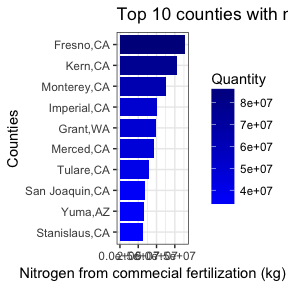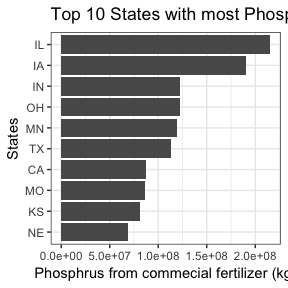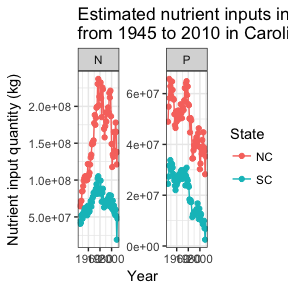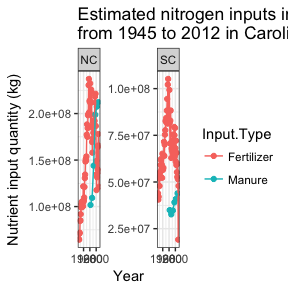# Introduction of usfertilizer, an R package

## Preface

Nutrients as commercial fertilizer is an important input to soil water systems, especially in agricultural fields. It is critical to, at least roughly, estimate the quantity of fertilizer application in the watershed, to conduct further evaluation of water quality from certain watersheds. Since 1990, folks from United States Geological Service (USGS) have investigated considerable time, manpower and efforts to estimate the fertilizer application at county scales in United States of America. Based on the sales data of commercial fertilizer, USGS researchers allocated the sold fertilizer to each county based on agricultural production, arable land, growing seasons, etc. Further details of data sources and specific attentions are available via https://wenlong-liu.github.io/usfertilizer/articles/Data_sources_and_cleaning.html.

Although there is no perfect way to estimate the nutrient application in watershed, the datasets from USGS have been carefully reviewed and can serve as an indication of nutrients input from commercial fertilizer and animal manure. In addition, please employ this datasets at watershed or regional scales. Please note that USGS does not endorse this package. Also data from 1986 is not available for now.

## Installment

You can also install it via CRAN:

install.packages("usfertilizer")

# Get started

## Summary of the dataset

The dataset, named by us_fertilizer_county, contains 625580 observations and 11 variables. Details are available by using ?us_fertilizer_county.

glimpse(us_fertilizer_county)
#> Observations: 625,580
#> Variables: 12
#> $FIPS <chr> "01001", "01003", "01005", "01007", "01009", "01011... #>$ State      <chr> "AL", "AL", "AL", "AL", "AL", "AL", "AL", "AL", "AL...
#> $County <chr> "Autauga", "Baldwin", "Barbour", "Bibb", "Blount", ... #>$ ALAND      <dbl> 1539582278, 4117521611, 2291818968, 1612480789, 166...
#> $AWATER <dbl> 25775735, 1133190229, 50864716, 9289057, 15157440, ... #>$ INTPTLAT   <dbl> 32.53638, 30.65922, 31.87067, 33.01589, 33.97745, 3...
#> $INTPTLONG <dbl> -86.64449, -87.74607, -85.40546, -87.12715, -86.567... #>$ Quantity   <dbl> 1580225, 6524369, 2412372, 304592, 1825118, 767573,...
#> $Year <chr> "1987", "1987", "1987", "1987", "1987", "1987", "19... #>$ Nutrient   <chr> "N", "N", "N", "N", "N", "N", "N", "N", "N", "N", "...
#> $Farm.Type <chr> "farm", "farm", "farm", "farm", "farm", "farm", "fa... #>$ Input.Type <chr> "Fertilizer", "Fertilizer", "Fertilizer", "Fertiliz...

## Examples

### Example 1: Find out the top 10 counties with most nitrogen appliation in 2008.

# plot the top 10 nitrogen application in year 2008.
# Reorder to make the plot more cleanner.
year_plot = 2008
us_fertilizer_county %>%
filter(Nutrient == "N" & Year == year_plot & Input.Type == "Fertilizer" ) %>%
top_n(10, Quantity) %>%
ggplot(aes(x=reorder(paste(County,State, sep = ","), Quantity), Quantity, fill = Quantity))+
scale_fill_gradient(low = "blue", high = "darkblue")+
geom_col()+
ggtitle(paste("Top 10 counties with most fertilizer application in the year of", year_plot)) +
scale_y_continuous(name = "Nitrogen from commecial fertilization (kg)")+
scale_x_discrete(name = "Counties")+
coord_flip()+
theme_bw()### Example 2: Find out the top 10 states with most nitrogen appliation in 1980.

# plot the top 10 states with P application in year 1980.
# Reorder to make the plot more cleanner.
year_plot = 1980
us_fertilizer_county %>%
filter(Nutrient == "P" & Year == 1980 & Input.Type == "Fertilizer") %>%
group_by(State) %>%
summarise(p_application = sum(Quantity)) %>%
as.data.frame() %>%
top_n(10, p_application) %>%
ggplot(aes(x=reorder(State, p_application), p_application))+
scale_fill_gradient(low = "blue", high = "darkblue")+
geom_col()+
ggtitle(paste("Top 10 States with most Phosphrus application in the year of", year_plot)) +
scale_y_continuous(name = "Phosphrus from commecial fertilizer (kg)")+
scale_x_discrete(name = "States")+
theme_bw()+
coord_flip()### Example 3: Plot the N and P input into farms for NC and SC from 1945 to 2010

year_plot = seq(1945, 2010, 1)
states = c("NC","SC")

us_fertilizer_county %>%
filter(State %in% states & Year %in% year_plot &
Farm.Type == "farm" & Input.Type == "Fertilizer") %>%
group_by(State, Year, Nutrient) %>%
summarise(Quantity = sum(Quantity, na.rm = T)) %>%
ggplot(aes(x = as.numeric(Year), y = Quantity, color=State)) +
geom_point() +
geom_line()+
scale_x_continuous(name = "Year")+
scale_y_continuous(name = "Nutrient input quantity (kg)")+
facet_wrap(~Nutrient, scales = "free", ncol = 2)+
ggtitle("Estimated nutrient inputs into arable lands by commercial fertilizer\nfrom 1945 to 2010 in Carolinas")+
theme_bw()### Example 4: Plot the N input into farms from fertilizer and manure for NC and SC from 1945 to 2012

us_fertilizer_county %>%
filter(State %in% states & Year %in% year_plot &
Farm.Type == "farm" & Nutrient == "N") %>%
group_by(State, Year, Input.Type) %>%
summarise(Quantity = sum(Quantity, na.rm = T)) %>%
ggplot(aes(x = as.numeric(Year), y = Quantity, color=Input.Type)) +
geom_point() +
geom_line()+
scale_x_continuous(name = "Year")+
scale_y_continuous(name = "Nutrient input quantity (kg)")+
facet_wrap(~State, scales = "free", ncol = 2)+
ggtitle("Estimated nitrogen inputs into arable lands by commercial fertilizer and manure\nfrom 1945 to 2012 in Carolinas")+
theme_bw()